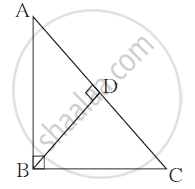Advertisement Remove all ads

# Prove That, in a Right Angled Triangle, the Square of the Hypotenuse is Equal to the Sum of the Squares of Remaining Two Sides. - Geometry

Prove that, in a right angled triangle, the square of the hypotenuse is
equal to the sum of the squares of remaining two sides.

Advertisement Remove all ads

#### SolutionGiven : In Δ ABC, ∠ ABC = 90o
To Prove : AC2 = AB2 + BC2
Construction : Draw seg BD perpendicular
on side AC. A-D-C.

Proof : In right angled Δ ABC,
seg BD ⊥ hypotenuse AC ...... (Construction)
∴ Δ ABC ∼ Δ ADB ∼ Δ BDC ...(Similarity of right angled triangles)
Δ ABC ∼ Δ ADB
∴(AB)/(AD)=(BC)/(DB)=(AC)/(AB)  .....(corresponding sides)
∴(AB)/(AD) =(AC)/(AB)
∴ AB2 = AD × AC ....... (I)
Δ ABC ∼ Δ BDC
(AB)/(BD) =(BC)/(DC) =(AC)/(BC)......(corresponding sides)

∴ (BC)/(DC) = (AC)/(BC)
∴ BC2 = AC × DC ....... (II)
Adding (I) and (II)
AB^2 + BC^2= AD × AC + AC × DC
∴ AB^2 + BC^2 = AC (AD + DC)
∴ AB^2 + BC^2 = AC × AC ...... (A-D-C)
∴ AB^2 + BC^2 = AC^2

Concept: Similarity in Right Angled Triangles
Is there an error in this question or solution?
Advertisement Remove all ads

#### Video TutorialsVIEW ALL 

Advertisement Remove all ads
Share
Notifications

View all notifications

Forgot password?